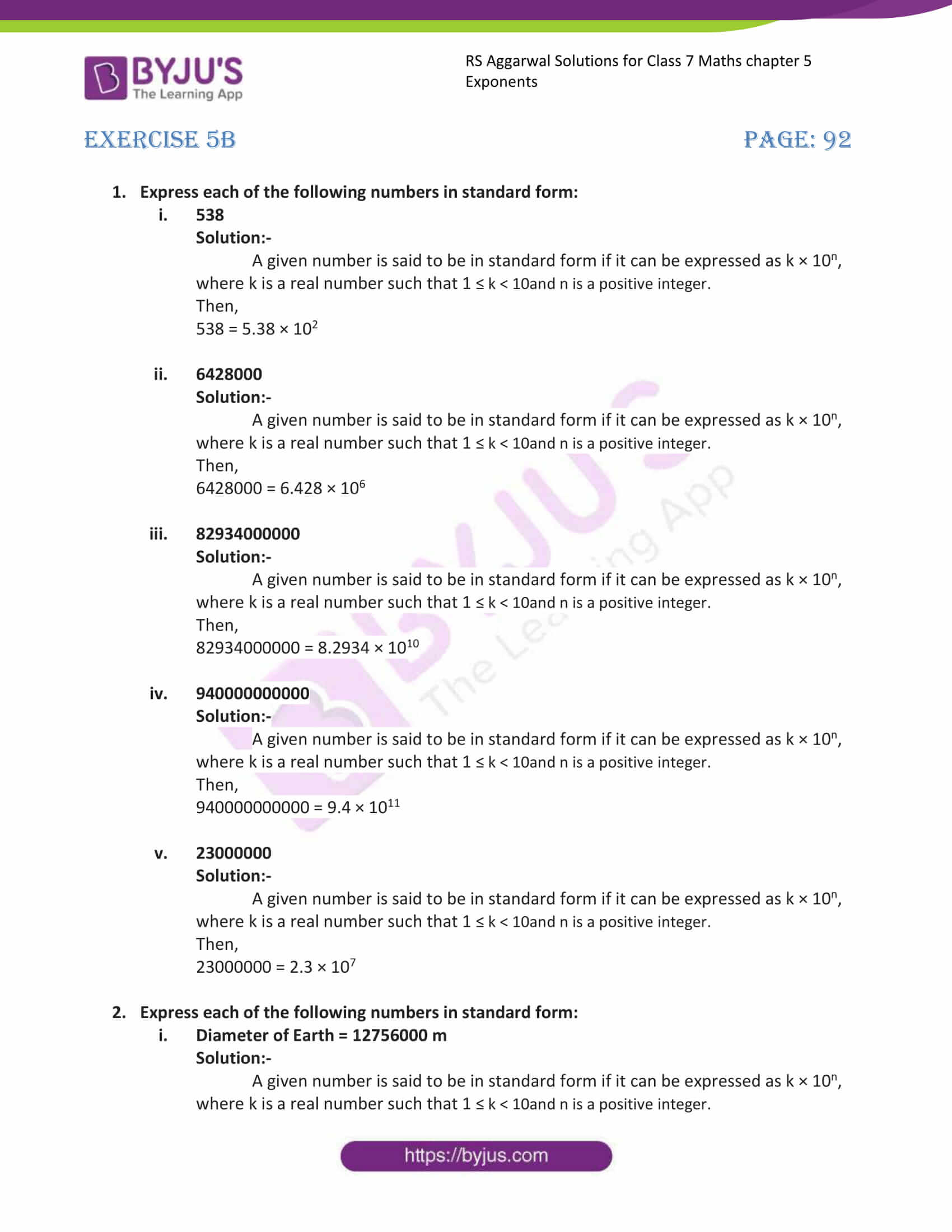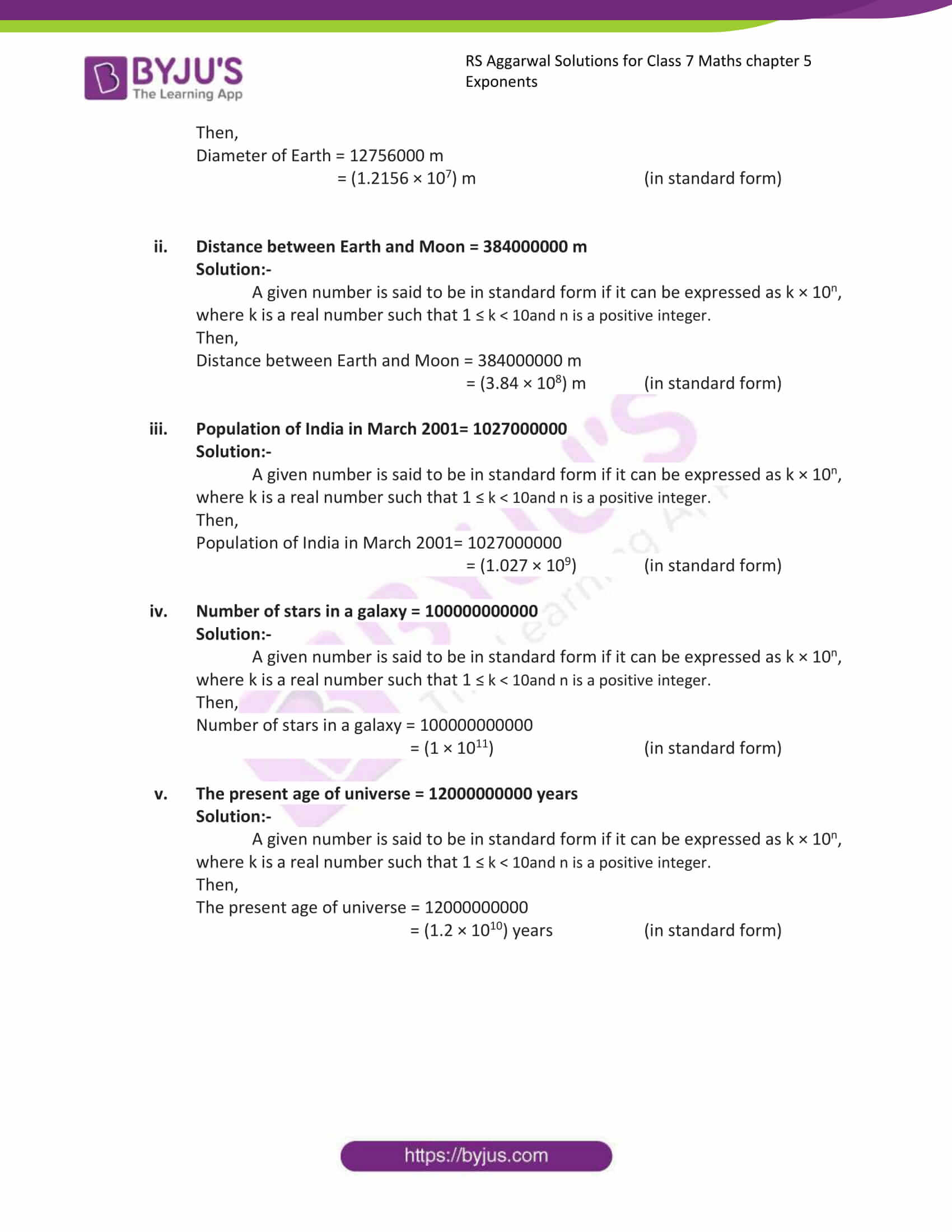# RS Aggarwal Solutions for Class 7 Maths Exercise 5B Chapter 5 Exponents

RS Aggarwal Solutions for Class 7 Maths Exercise 5B Chapter 5 Exponents, the RS Aggarwal is an essential material as it offers a wide range of questions that test the students understanding of concepts. This exercise contains expressing large numbers in standard form; a given number is said to be standard form if it can be expressed as k×(10 to the power of n). Our expert personnel have solved the problems step by step with neat explanations. Students can download the PDF of RS Aggarwal Solutions for Class 7 Maths Chapter 5 Exponents. Students gain more knowledge by referring to RS Aggarwal Class 7 Solutions.

## Download the PDF of RS Aggarwal Solutions For Class 7 Maths Chapter 5 Exponents – Exercise 5B### Access answers to Maths RS Aggarwal Solutions for Class 7 Chapter 5 – Exponents Exercise 5B

1. Express each of the following numbers in standard form:

(i) 538

Solution:-

A given number is said to be in standard form if it can be expressed as k × 10n, where k is a real number such that 1 ≤ k < 10and n is a positive integer.

Then,

538 = 5.38 × 102

(ii) 6428000

Solution:-

A given number is said to be in standard form if it can be expressed as k × 10n, where k is a real number such that 1 ≤ k < 10and n is a positive integer.

Then,

6428000 = 6.428 × 106

(iii) 82934000000

Solution:-

A given number is said to be in standard form if it can be expressed as k × 10n, where k is a real number such that 1 ≤ k < 10and n is a positive integer.

Then,

82934000000 = 8.2934 × 1010

(iv) 940000000000

Solution:-

A given number is said to be in standard form if it can be expressed as k × 10n, where k is a real number such that 1 ≤ k < 10and n is a positive integer.

Then,

940000000000 = 9.4 × 1011

(v) 23000000

Solution:-

A given number is said to be in standard form if it can be expressed as k × 10n, where k is a real number such that 1 ≤ k < 10and n is a positive integer.

Then,

23000000 = 2.3 × 107

2. Express each of the following numbers in standard form:

(i) Diameter of Earth = 12756000 m

Solution:-

A given number is said to be in standard form if it can be expressed as k × 10n, where k is a real number such that 1 ≤ k < 10and n is a positive integer.

Then,

Diameter of Earth = 12756000 m

= (1.2156 × 107) m (in standard form)

(ii) Distance between Earth and Moon = 384000000 m

Solution:-

A given number is said to be in standard form if it can be expressed as k × 10n, where k is a real number such that 1 ≤ k < 10and n is a positive integer.

Then,

Distance between Earth and Moon = 384000000 m

= (3.84 × 108) m (in standard form)

(iii) Population of India in March 2001= 1027000000

Solution:-

A given number is said to be in standard form if it can be expressed as k × 10n, where k is a real number such that 1 ≤ k < 10and n is a positive integer.

Then,

Population of India in March 2001= 1027000000

= (1.027 × 109) (in standard form)

(iv) Number of stars in a galaxy = 100000000000

Solution:-

A given number is said to be in standard form if it can be expressed as k × 10n, where k is a real number such that 1 ≤ k < 10and n is a positive integer.

Then,

Number of stars in a galaxy = 100000000000

= (1 × 1011) (in standard form)

(v) The present age of universe = 12000000000 years

Solution:-

A given number is said to be in standard form if it can be expressed as k × 10n, where k is a real number such that 1 ≤ k < 10and n is a positive integer.

Then,

The present age of universe = 12000000000

= (1.2 × 1010) years (in standard form)

### Access other exercises of RS Aggarwal Solutions For Class 7 Chapter 5 – Exponents

Exercise 5C Solutions